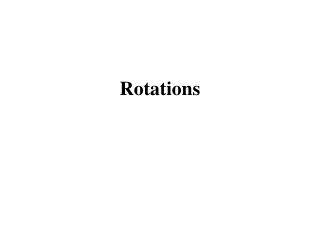# Rotations - PowerPoint PPT PresentationDownload PresentationRotations

RotationsDownload Presentation## Rotations

- - - - - - - - - - - - - - - - - - - - - - - - - - - E N D - - - - - - - - - - - - - - - - - - - - - - - - - - -
##### Presentation Transcript

1. Rotations

2. Goal • Identify rotations and rotational symmetry.

3. Key Vocabulary • Rotation • Center of rotation • Angle of rotation • Rotational symmetry

4. Rotation Vocabulary • Rotation – transformation that turns every point of a pre-image through a specified angle and direction about a fixed point. image Pre-image rotation fixed point

5. Rotation Vocabulary • Center of rotation – fixed point of the rotation. Center of Rotation

6. Rotation Vocabulary • Angle of rotation – angle between a pre-image point and corresponding image point. image Pre-image Angle of Rotation

7. Example: Click the triangle to see rotation Center of Rotation Rotation

8. Example 1: Identifying Rotations Tell whether each transformation appears to be a rotation. Explain. B. A. No; the figure appears to be flipped. Yes; the figure appears to be turned around a point.

9. Your Turn: Tell whether each transformation appears to be a rotation. b. a. Yes, the figure appears to be turned around a point. No, the figure appears to be a translation.

10. Rotation Vocabulary • Rotational symmetry – A figure in a plane has rotational symmetry if the figure can be mapped onto itself by a rotation of 180⁰ or less. Has rotational symmetry because it maps onto itself by a rotation of 90⁰.

11. Equilateral Triangle An equilateral triangle has rotational symmetry of order ?

12. Equilateral Triangle An equilateral triangle has rotational symmetry of order ?

13. Equilateral Triangle 3 An equilateral triangle has rotational symmetry of order ? 3 2 1

14. Hexagon Regular Hexagon A regular hexagon has rotational symmetry of order ?

15. Regular Hexagon A regular hexagon has rotational symmetry of order ?

16. Regular Hexagon 6 A regular hexagon has rotational symmetry of order ? 5 6 1 4 2 3

17. Rotational Symmetry Whena figure can be rotated less than 360° and the image and pre-image are indistinguishable (regular polygons are a great example). Symmetry Rotational: 120° 90° 60° 45°

18. a. Rectangle b. Regular hexagon c. Trapezoid SOLUTION a. Yes. A rectangle can be mapped onto itself by a clockwise or counterclockwise rotation of 180° about its center. Example 2 Identify Rotational Symmetry Does the figure have rotational symmetry? If so, describe the rotations that map the figure onto itself.

19. b. Yes. A regular hexagon can be mapped onto itself by a clockwise or counterclockwise rotation of 60°, 120°, or 180° about its center. c. No. A trapezoid does not have rotational symmetry. Example 2 Identify Rotational Symmetry Regular hexagon Trapezoid

20. Your Turn: 1. Isosceles trapezoid no ANSWER 2. Parallelogram yes; a clockwise or counterclockwise rotation of 180° about its center ANSWER Does the figure have rotational symmetry? If so, describe the rotations that map the figure onto itself.

21. Your Turn: 3. Regular octagon yes; a clockwise or counterclockwise rotation of 45°, 90°, 135°, or 180° about its center ANSWER

22. Rotation in a Coordinate Plane • For a Rotation, you need; • An angle or degree of turn • Eg 90° or a Quarter Turn • E.g. 180 ° or a Half Turn • A direction • Clockwise • Anticlockwise • A Centre of Rotation • A point around which Object rotates

23. 8 7 6 y 5 4 3 2 1 x x x x 1 2 3 4 5 6 7 8 –7 –6 –5 –4 –3 –2 –1 -1 x -2 -3 -4 -5 -6 x A Rotation of 90° Counterclockwiseabout (0,0) (x, y)→(-y, x) C(3,5) B’(-2,4) C’(-5,3) B(4,2) A’(-1,2) A(2,1)

24. 8 7 6 y 5 4 3 2 1 x x x x x x x 1 2 3 4 5 6 7 8 –7 –6 –5 –4 –3 –2 –1 -1 x -2 -3 -4 -5 -6 x A Rotation of 180° about (0,0) (x, y)→(-x, -y) C(3,5) B(4,2) A(2,1) A’(-2,-1) B’(-4,-2) C’(-3,-5)

25. Rotation in a Coordinate Plane

26. SOLUTION Plot the points, as shown in blue. Example 4 Rotations in a Coordinate Plane Sketch the quadrilateral with vertices A(2, –2),B(4, 1), C(5, 1), andD(5, –1). Rotate it 90° counterclockwise about the origin and name the coordinates of the new vertices. Use a protractor and a ruler to find the rotated vertices. The coordinates of the vertices of the image are A'(2, 2),B'(–1, 4), C'(–1, 5),andD'(1, 5).

27. Checkpoint 4. Sketch the triangle with vertices A(0, 0),B(3, 0),andC(3, 4).Rotate∆ABC 90°counterclockwise about the origin. Name the coordinates of the new vertices A',B', and C'. ANSWER Rotations in a Coordinate Plane • A'(0,0), B'(0, 3), C'(–4, 3)